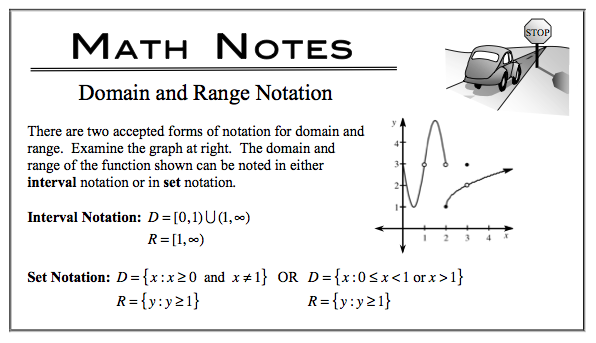### Home > CALC > Chapter 1 > Lesson 1.2.6 > Problem1-94

1-94.
1. Given f(x) = (x − 3)2 + 4 and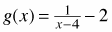, find and simplify the following: Homework Help ✎

1. h(x)= f(x + 2) − 5

2. k(x) = g(3 − x) + 2

3. Using set notation, find the domain and range for g(x) and k(x).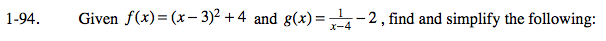h(x) = f(x + 2) = 5
h(x) = [((x + 2) − 3) 2] + 4 − 5
Simplify.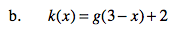(3 − x), the horizontal shift, will replace 'x' in the g(x) function.
+2, the vertical shift, will be added to the entire 'g(x)' function.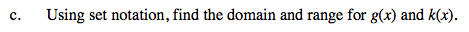Consider the parent graphs of f and g.
Domain and range will shift from that of the parent.
The parent graph of f(x) is y = x².
What is the parent graph of g(x)?